# Napp-It not scaling well ... - revisited ;)

#### Rand__

##### Well-Known Member
So,
2 years later (https://forums.servethehome.com/index.php?threads/napp-it-not-scaling-well.17154/) still the same problems :/

O/c I have to say its not Napp-It, its ZFS; this time on OmniOS latest (but similar problems on latest FreeNas).

I have a Xeon Gold 6150 QS (2.7GHz base, all core freq 3.4GHz iirc), and 13 HGST SS300 SAS3 drives to play with (800GB). Those drives are attached to two 9305-16i's, 6 drives each; o/c on PCIe3-x8 slots on a single CPU Board (X11SPH-nCTPF); running in a CSE-216 with -A backplane.

I have installed fio to run tests, using the following command to run a pure seq write test:

/opt/csw/bin/fio --refill_buffers --norandommap --randrepeat=0 --group_reporting --ioengine=solarisaio --name="<testname>" --runtime=60 --size=100G --time_based --bs=128k --iodepth=1 --numjobs=1 --rw=write --filename=<outfile>

I have pools with a single disk up to 6 disks (striped) and then 13 disks; a dataset with sync off, no compression, no atime and blocksize 128k.

These tests were explicitly done to see how fast a pool can go for a single thread (which is not very fast at this time for yet unknown reasons).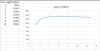CPU load (using prstat) peaked at 3.7% (as always its not clear to me whether that's 3.7% of a single core or of total capacity [The physical processor has 18 cores and 36 virtual processors (0-35)]).

So as one can see: a single disk is able to do 900 MB/s writes, adding a second increases by 300 MB/s, a third by another 100 and adding a fourth disk is basically useless.

Below are the detailed testruns for OmniOS
Code:
``````/opt/csw/bin/fio   --refill_buffers --norandommap --randrepeat=0 --group_reporting --ioengine=solarisaio --name="stripe1/ds1_128k_stripe1"  --runtime=60 --size=100G --time_based  --bs=128k --iodepth=1 --numjobs=1 --rw=write --filename=/stripe1/ds1/fio_1.out
stripe1/ds1_128k: (g=0): rw=write, bs=128K-128K/128K-128K/128K-128K, ioengine=solarisaio, iodepth=1
fio-2.0.14
Starting 1 process
stripe1/ds1_128k: Laying out IO file(s) (1 file(s) / 102400MB)
Jobs: 1 (f=1): [W] [100.0% done] [0K/990.2M/0K /s] [0 /7921 /0  iops] [eta 00m:00s]
stripe1/ds1_128k: (groupid=0, jobs=1): err= 0: pid=5929: Wed Nov 20 22:33:13 2019
write: io=911488KB, bw=923957KB/s, iops=7218 , runt= 60000msec
slat (usec): min=2 , max=366 , avg= 2.84, stdev= 0.79
clat (usec): min=29 , max=258912 , avg=86.68, stdev=487.77
lat (usec): min=33 , max=258915 , avg=89.52, stdev=487.77
clat percentiles (usec):
|  1.00th=[   33],  5.00th=[   35], 10.00th=[   35], 20.00th=[   36],
| 30.00th=[   39], 40.00th=[   51], 50.00th=[   57], 60.00th=[   69],
| 70.00th=[   86], 80.00th=[   93], 90.00th=[  147], 95.00th=[  245],
| 99.00th=[  470], 99.50th=[  524], 99.90th=[  644], 99.95th=[  724],
| 99.99th=
bw (KB/s)  : min=252672, max=1343488, per=100.00%, avg=926805.27, stdev=248607.24
lat (usec) : 50=39.14%, 100=44.00%, 250=12.02%, 500=4.15%, 750=0.65%
lat (usec) : 1000=0.01%
lat (msec) : 2=0.01%, 4=0.01%, 10=0.01%, 20=0.01%, 50=0.01%
lat (msec) : 100=0.01%, 500=0.01%
cpu          : usr=106.99%, sys=55.18%, ctx=449288, majf=0, minf=0
IO depths    : 1=100.0%, 2=0.0%, 4=0.0%, 8=0.0%, 16=0.0%, 32=0.0%, >=64=0.0%
submit    : 0=0.0%, 4=100.0%, 8=0.0%, 16=0.0%, 32=0.0%, 64=0.0%, >=64=0.0%
complete  : 0=0.0%, 4=100.0%, 8=0.0%, 16=0.0%, 32=0.0%, 64=0.0%, >=64=0.0%
issued    : total=r=0/w=0/d=433105, short=r=0/w=0/d=0

Run status group 0 (all jobs):
WRITE: io=54138MB, aggrb=923957KB/s, minb=923957KB/s, maxb=923957KB/s, mint=60000msec, maxt=60000msec

/opt/csw/bin/fio   --refill_buffers --norandommap --randrepeat=0 --group_reporting --ioengine=solarisaio --name="stripe1/ds1_128k_stripe2"  --runtime=60 --size=100G --time_based  --bs=128k --iodepth=1 --numjobs=1 --rw=write --filename=/stripe1/ds1/fio_1.out
stripe1/ds1_128k: (g=0): rw=write, bs=128K-128K/128K-128K/128K-128K, ioengine=solarisaio, iodepth=1
fio-2.0.14
Starting 1 process
stripe1/ds1_128k: Laying out IO file(s) (1 file(s) / 102400MB)
Jobs: 1 (f=1): [W] [100.0% done] [0K/908.9M/0K /s] [0 /7271 /0  iops] [eta 00m:00s]
stripe1/ds1_128k: (groupid=0, jobs=1): err= 0: pid=6416: Wed Nov 20 22:35:12 2019
write: io=1929.2MB, bw=1192.7MB/s, iops=9541 , runt= 60001msec
slat (usec): min=2 , max=381 , avg= 2.76, stdev= 0.77
clat (usec): min=28 , max=20885 , avg=52.99, stdev=107.18
lat (usec): min=32 , max=20887 , avg=55.75, stdev=107.20
clat percentiles (usec):
|  1.00th=[   32],  5.00th=[   34], 10.00th=[   35], 20.00th=[   35],
| 30.00th=[   36], 40.00th=[   37], 50.00th=[   38], 60.00th=[   40],
| 70.00th=[   45], 80.00th=[   56], 90.00th=[   86], 95.00th=[   91],
| 99.00th=[  225], 99.50th=[  438], 99.90th=[  828], 99.95th=[  916],
| 99.99th=[ 1608]
bw (MB/s)  : min=  677, max= 1398, per=100.00%, avg=1224.04, stdev=229.13
lat (usec) : 50=75.25%, 100=21.38%, 250=2.46%, 500=0.49%, 750=0.26%
lat (usec) : 1000=0.12%
lat (msec) : 2=0.02%, 4=0.01%, 20=0.01%, 50=0.01%
cpu          : usr=106.54%, sys=43.56%, ctx=593789, majf=0, minf=0
IO depths    : 1=100.0%, 2=0.0%, 4=0.0%, 8=0.0%, 16=0.0%, 32=0.0%, >=64=0.0%
submit    : 0=0.0%, 4=100.0%, 8=0.0%, 16=0.0%, 32=0.0%, 64=0.0%, >=64=0.0%
complete  : 0=0.0%, 4=100.0%, 8=0.0%, 16=0.0%, 32=0.0%, 64=0.0%, >=64=0.0%
issued    : total=r=0/w=0/d=572489, short=r=0/w=0/d=0

Run status group 0 (all jobs):
WRITE: io=71561MB, aggrb=1192.7MB/s, minb=1192.7MB/s, maxb=1192.7MB/s, mint=60001msec, maxt=60001msec

zpool status stripe1
pool: stripe1
state: ONLINE
scan: none requested
config:

stripe1                   ONLINE       0     0     0
c0t5000CCA082001CE4d0   ONLINE       0     0     0
c10t5000CCA082006ED1d0  ONLINE       0     0     0
c11t5000CCA082007151d0  ONLINE       0     0     0

errors: No known data errors

/opt/csw/bin/fio   --refill_buffers --norandommap --randrepeat=0 --group_reporting --ioengine=solarisaio --name="stripe1/ds1_128k_stripe3"  --runtime=60 --size=100G --time_based  --bs=128k --iodepth=1 --numjobs=1 --rw=write --filename=/stripe1/ds1/fio_1.out
stripe1/ds1_128k_stripe3: (g=0): rw=write, bs=128K-128K/128K-128K/128K-128K, ioengine=solarisaio, iodepth=1
fio-2.0.14
Starting 1 process
stripe1/ds1_128k_stripe3: Laying out IO file(s) (1 file(s) / 102400MB)
Jobs: 1 (f=1): [W] [100.0% done] [0K/899.2M/0K /s] [0 /7193 /0  iops] [eta 00m:00s]
stripe1/ds1_128k_stripe3: (groupid=0, jobs=1): err= 0: pid=7028: Wed Nov 20 22:38:02 2019
write: io=126208KB, bw=1299.2MB/s, iops=10392 , runt= 60001msec
slat (usec): min=2 , max=399 , avg= 2.81, stdev= 0.76
clat (usec): min=5 , max=19696 , avg=44.36, stdev=70.88
lat (usec): min=31 , max=19701 , avg=47.16, stdev=70.91
clat percentiles (usec):
|  1.00th=[   33],  5.00th=[   35], 10.00th=[   35], 20.00th=[   36],
| 30.00th=[   36], 40.00th=[   37], 50.00th=[   37], 60.00th=[   39],
| 70.00th=[   41], 80.00th=[   46], 90.00th=[   57], 95.00th=[   84],
| 99.00th=[   95], 99.50th=[  101], 99.90th=[  239], 99.95th=[ 1080],
| 99.99th=[ 2640]
bw (MB/s)  : min=  735, max= 1462, per=100.00%, avg=1332.60, stdev=159.71
lat (usec) : 10=0.01%, 50=84.49%, 100=14.92%, 250=0.49%, 500=0.02%
lat (usec) : 750=0.02%, 1000=0.01%
lat (msec) : 2=0.03%, 4=0.02%, 10=0.01%, 20=0.01%
cpu          : usr=105.56%, sys=40.03%, ctx=648325, majf=0, minf=0
IO depths    : 1=100.0%, 2=0.0%, 4=0.0%, 8=0.0%, 16=0.0%, 32=0.0%, >=64=0.0%
submit    : 0=0.0%, 4=100.0%, 8=0.0%, 16=0.0%, 32=0.0%, 64=0.0%, >=64=0.0%
complete  : 0=0.0%, 4=100.0%, 8=0.0%, 16=0.0%, 32=0.0%, 64=0.0%, >=64=0.0%
issued    : total=r=0/w=0/d=623578, short=r=0/w=0/d=0

Run status group 0 (all jobs):
WRITE: io=77947MB, aggrb=1299.2MB/s, minb=1299.2MB/s, maxb=1299.2MB/s, mint=60001msec, maxt=60001msec

zpool status stripe1
pool: stripe1
state: ONLINE
scan: none requested
config:

stripe1                   ONLINE       0     0     0
c0t5000CCA082001CE4d0   ONLINE       0     0     0
c10t5000CCA082006ED1d0  ONLINE       0     0     0
c11t5000CCA082007151d0  ONLINE       0     0     0
c12t5000CCA082006751d0  ONLINE       0     0     0

errors: No known data errors
root@omniosce:~# /opt/csw/bin/fio   --refill_buffers --norandommap --randrepeat=0 --group_reporting --ioengine=solarisaio --name="stripe1/ds1_128k_stripe4"  --runtime=60 --size=100G --time_based  --bs=128k --iodepth=1 --numjobs=1 --rw=write --filename=/stripe1/ds1/fio_1.out
stripe1/ds1_128k_stripe4: (g=0): rw=write, bs=128K-128K/128K-128K/128K-128K, ioengine=solarisaio, iodepth=1
fio-2.0.14
Starting 1 process
stripe1/ds1_128k_stripe4: Laying out IO file(s) (1 file(s) / 102400MB)
Jobs: 1 (f=1): [W] [100.0% done] [0K/1165M/0K /s] [0 /9322 /0  iops] [eta 00m:00s]
stripe1/ds1_128k_stripe4: (groupid=0, jobs=1): err= 0: pid=7409: Wed Nov 20 22:39:44 2019
write: io=1171.2MB, bw=1316.6MB/s, iops=10532 , runt= 60001msec
slat (usec): min=2 , max=395 , avg= 2.80, stdev= 0.75
clat (usec): min=28 , max=14262 , avg=43.10, stdev=33.82
lat (usec): min=32 , max=14264 , avg=45.90, stdev=33.85
clat percentiles (usec):
|  1.00th=[   33],  5.00th=[   34], 10.00th=[   35], 20.00th=[   36],
| 30.00th=[   36], 40.00th=[   37], 50.00th=[   37], 60.00th=[   39],
| 70.00th=[   42], 80.00th=[   46], 90.00th=[   56], 95.00th=[   69],
| 99.00th=[  103], 99.50th=[  129], 99.90th=[  197], 99.95th=[  219],
| 99.99th=[  454]
bw (MB/s)  : min=  991, max= 1446, per=100.00%, avg=1353.19, stdev=50.85
lat (usec) : 50=84.09%, 100=14.76%, 250=1.12%, 500=0.02%, 750=0.01%
lat (usec) : 1000=0.01%
lat (msec) : 2=0.01%, 4=0.01%, 10=0.01%, 20=0.01%
cpu          : usr=107.77%, sys=37.08%, ctx=657156, majf=0, minf=0
IO depths    : 1=100.0%, 2=0.0%, 4=0.0%, 8=0.0%, 16=0.0%, 32=0.0%, >=64=0.0%
submit    : 0=0.0%, 4=100.0%, 8=0.0%, 16=0.0%, 32=0.0%, 64=0.0%, >=64=0.0%
complete  : 0=0.0%, 4=100.0%, 8=0.0%, 16=0.0%, 32=0.0%, 64=0.0%, >=64=0.0%
issued    : total=r=0/w=0/d=631961, short=r=0/w=0/d=0

Run status group 0 (all jobs):
WRITE: io=78995MB, aggrb=1316.6MB/s, minb=1316.6MB/s, maxb=1316.6MB/s, mint=60001msec, maxt=60001msec

zpool status stripe1
pool: stripe1
state: ONLINE
scan: none requested
config:

stripe1                   ONLINE       0     0     0
c0t5000CCA082001CE4d0   ONLINE       0     0     0
c10t5000CCA082006ED1d0  ONLINE       0     0     0
c11t5000CCA082007151d0  ONLINE       0     0     0
c12t5000CCA082006751d0  ONLINE       0     0     0
c13t5000CCA082007279d0  ONLINE       0     0     0

errors: No known data errors

/opt/csw/bin/fio   --refill_buffers --norandommap --randrepeat=0 --group_reporting --ioengine=solarisaio --name="stripe1/ds1_128k_stripe5"  --runtime=60 --size=100G --time_based  --bs=128k --iodepth=1 --numjobs=1 --rw=write --filename=/stripe1/ds1/fio_1.out
stripe1/ds1_128k_stripe5: (g=0): rw=write, bs=128K-128K/128K-128K/128K-128K, ioengine=solarisaio, iodepth=1
fio-2.0.14
Starting 1 process
stripe1/ds1_128k_stripe5: Laying out IO file(s) (1 file(s) / 102400MB)
Jobs: 1 (f=1): [W] [100.0% done] [0K/873.3M/0K /s] [0 /6986 /0  iops] [eta 00m:00s]
stripe1/ds1_128k_stripe5: (groupid=0, jobs=1): err= 0: pid=7832: Wed Nov 20 22:41:40 2019
write: io=2484.4MB, bw=1338.5MB/s, iops=10707 , runt= 60001msec
slat (usec): min=2 , max=367 , avg= 2.86, stdev= 0.70
clat (usec): min=28 , max=13956 , avg=41.46, stdev=25.17
lat (usec): min=31 , max=13959 , avg=44.32, stdev=25.21
clat percentiles (usec):
|  1.00th=[   32],  5.00th=[   34], 10.00th=[   35], 20.00th=[   36],
| 30.00th=[   36], 40.00th=[   37], 50.00th=[   37], 60.00th=[   38],
| 70.00th=[   40], 80.00th=[   44], 90.00th=[   52], 95.00th=[   62],
| 99.00th=[   88], 99.50th=[   95], 99.90th=[  129], 99.95th=[  153],
| 99.99th=[  318]
bw (MB/s)  : min=  809, max= 1504, per=100.00%, avg=1373.90, stdev=63.18
lat (usec) : 50=87.35%, 100=12.27%, 250=0.37%, 500=0.01%, 750=0.01%
lat (usec) : 1000=0.01%
lat (msec) : 2=0.01%, 4=0.01%, 20=0.01%
cpu          : usr=105.91%, sys=37.94%, ctx=667700, majf=0, minf=0
IO depths    : 1=100.0%, 2=0.0%, 4=0.0%, 8=0.0%, 16=0.0%, 32=0.0%, >=64=0.0%
submit    : 0=0.0%, 4=100.0%, 8=0.0%, 16=0.0%, 32=0.0%, 64=0.0%, >=64=0.0%
complete  : 0=0.0%, 4=100.0%, 8=0.0%, 16=0.0%, 32=0.0%, 64=0.0%, >=64=0.0%
issued    : total=r=0/w=0/d=642467, short=r=0/w=0/d=0

Run status group 0 (all jobs):
WRITE: io=80308MB, aggrb=1338.5MB/s, minb=1338.5MB/s, maxb=1338.5MB/s, mint=60001msec, maxt=60001msec

zpool status stripe1
pool: stripe1
state: ONLINE
scan: none requested
config:

stripe1                   ONLINE       0     0     0
c0t5000CCA082001CE4d0   ONLINE       0     0     0
c10t5000CCA082006ED1d0  ONLINE       0     0     0
c11t5000CCA082007151d0  ONLINE       0     0     0
c12t5000CCA082006751d0  ONLINE       0     0     0
c13t5000CCA082007279d0  ONLINE       0     0     0
c14t5000CCA082006749d0  ONLINE       0     0     0

errors: No known data errors
root@omniosce:~# /opt/csw/bin/fio   --refill_buffers --norandommap --randrepeat=0 --group_reporting --ioengine=solarisaio --name="stripe1/ds1_128k_stripe6"  --runtime=60 --size=100G --time_based  --bs=128k --iodepth=1 --numjobs=1 --rw=write --filename=/stripe1/ds1/fio_1.out
stripe1/ds1_128k_stripe6: (g=0): rw=write, bs=128K-128K/128K-128K/128K-128K, ioengine=solarisaio, iodepth=1
fio-2.0.14
Starting 1 process
stripe1/ds1_128k_stripe6: Laying out IO file(s) (1 file(s) / 102400MB)
Jobs: 1 (f=1): [W] [100.0% done] [0K/1356M/0K /s] [0 /10.9K/0  iops] [eta 00m:00s]
stripe1/ds1_128k_stripe6: (groupid=0, jobs=1): err= 0: pid=8263: Wed Nov 20 22:43:36 2019
write: io=2632.8MB, bw=1340.1MB/s, iops=10727 , runt= 60000msec
slat (usec): min=2 , max=367 , avg= 2.80, stdev= 0.72
clat (usec): min=29 , max=619 , avg=41.37, stdev=12.57
lat (usec): min=32 , max=621 , avg=44.17, stdev=12.67
clat percentiles (usec):
|  1.00th=[   33],  5.00th=[   35], 10.00th=[   35], 20.00th=[   36],
| 30.00th=[   36], 40.00th=[   36], 50.00th=[   37], 60.00th=[   38],
| 70.00th=[   40], 80.00th=[   44], 90.00th=[   53], 95.00th=[   63],
| 99.00th=[   92], 99.50th=[  107], 99.90th=[  169], 99.95th=[  195],
| 99.99th=[  266]
bw (MB/s)  : min= 1327, max= 1429, per=100.00%, avg=1374.82, stdev=20.00
lat (usec) : 50=87.03%, 100=12.29%, 250=0.66%, 500=0.01%, 750=0.01%
cpu          : usr=106.23%, sys=37.57%, ctx=670686, majf=0, minf=0
IO depths    : 1=100.0%, 2=0.0%, 4=0.0%, 8=0.0%, 16=0.0%, 32=0.0%, >=64=0.0%
submit    : 0=0.0%, 4=100.0%, 8=0.0%, 16=0.0%, 32=0.0%, 64=0.0%, >=64=0.0%
complete  : 0=0.0%, 4=100.0%, 8=0.0%, 16=0.0%, 32=0.0%, 64=0.0%, >=64=0.0%
issued    : total=r=0/w=0/d=643654, short=r=0/w=0/d=0

Run status group 0 (all jobs):
WRITE: io=80457MB, aggrb=1340.1MB/s, minb=1340.1MB/s, maxb=1340.1MB/s, mint=60000msec, maxt=60000msec

zpool status stripe1
pool: stripe1
state: ONLINE
scan: none requested
config:

stripe1                   ONLINE       0     0     0
c0t5000CCA082001CE4d0   ONLINE       0     0     0
c10t5000CCA082006ED1d0  ONLINE       0     0     0
c11t5000CCA082007151d0  ONLINE       0     0     0
c12t5000CCA082006751d0  ONLINE       0     0     0
c13t5000CCA082007279d0  ONLINE       0     0     0
c14t5000CCA082006749d0  ONLINE       0     0     0
c15t5000CCA082006E85d0  ONLINE       0     0     0
c16t5000CCA082007059d0  ONLINE       0     0     0
c17t5000CCA082007339d0  ONLINE       0     0     0
c18t5000CCA082007355d0  ONLINE       0     0     0
c19t5000CCA082007335d0  ONLINE       0     0     0
c8t5000CCA08200733Dd0   ONLINE       0     0     0
c9t5000CCA08200734Dd0   ONLINE       0     0     0

errors: No known data errors
root@omniosce:~# /opt/csw/bin/fio   --refill_buffers --norandommap --randrepeat=0 --group_reporting --ioengine=solarisaio --name="stripe1/ds1_128k_stripe13"  --runtime=60 --size=100G --time_based  --bs=128k --iodepth=1 --numjobs=1 --rw=write --filename=/stripe1/ds1/fio_1.out
stripe1/ds1_128k_stripe13: (g=0): rw=write, bs=128K-128K/128K-128K/128K-128K, ioengine=solarisaio, iodepth=1
fio-2.0.14
Starting 1 process
stripe1/ds1_128k_stripe13: Laying out IO file(s) (1 file(s) / 102400MB)
Jobs: 1 (f=1): [W] [100.0% done] [0K/1345M/0K /s] [0 /10.8K/0  iops] [eta 00m:00s]
stripe1/ds1_128k_stripe13: (groupid=0, jobs=1): err= 0: pid=9091: Wed Nov 20 22:47:57 2019
write: io=75776KB, bw=1298.3MB/s, iops=10386 , runt= 60001msec
slat (usec): min=2 , max=392 , avg= 2.85, stdev= 0.85
clat (usec): min=28 , max=7672 , avg=44.35, stdev=32.81
lat (usec): min=32 , max=7678 , avg=47.19, stdev=33.03
clat percentiles (usec):
|  1.00th=[   32],  5.00th=[   34], 10.00th=[   35], 20.00th=[   35],
| 30.00th=[   36], 40.00th=[   36], 50.00th=[   37], 60.00th=[   38],
| 70.00th=[   40], 80.00th=[   45], 90.00th=[   58], 95.00th=[   78],
| 99.00th=[  147], 99.50th=[  195], 99.90th=[  430], 99.95th=[  628],
| 99.99th=[  980]
bw (MB/s)  : min= 1244, max= 1498, per=100.00%, avg=1329.82, stdev=42.81
lat (usec) : 50=85.19%, 100=12.07%, 250=2.47%, 500=0.20%, 750=0.04%
lat (usec) : 1000=0.02%
lat (msec) : 2=0.01%, 4=0.01%, 10=0.01%
cpu          : usr=107.78%, sys=36.27%, ctx=649328, majf=0, minf=0
IO depths    : 1=100.0%, 2=0.0%, 4=0.0%, 8=0.0%, 16=0.0%, 32=0.0%, >=64=0.0%
submit    : 0=0.0%, 4=100.0%, 8=0.0%, 16=0.0%, 32=0.0%, 64=0.0%, >=64=0.0%
complete  : 0=0.0%, 4=100.0%, 8=0.0%, 16=0.0%, 32=0.0%, 64=0.0%, >=64=0.0%
issued    : total=r=0/w=0/d=623184, short=r=0/w=0/d=0

Run status group 0 (all jobs):
WRITE: io=77898MB, aggrb=1298.3MB/s, minb=1298.3MB/s, maxb=1298.3MB/s, mint=60001msec, maxt=60001msec``````

Now the behavior is similar with other blocksizes, see this run from FreeNas with 1M recordsize/ fio testsize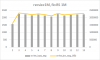and similar results are there for the other end (recordsize 4k, fio blocksize 4k, ~250 MB/s)

Now of course the question is why ?Can anyone confirm this behavior or dispute it?

#### T_Minus

##### Build. Break. Fix. Repeat
more disks = more latency
= It doesn't matter if those disks are the best in the world your test is doing something specific and limiting them ie: 1 JOB, 1 Depth.

This test seems to prove that I JOB @ 1 Depth is limited to this.

What occurs if you increase to 2 jobs?
Then 2 jobs and 2 depth?

Does performance increase?

#### gea

##### Well-Known Member
ZFS is called the most advanced filesystem on earth. This is true for sure but what you expect from it is was never its design goal.

If you have an Optane with 500k iops and 2GB/s throughput and stripe it with n others, you want a scaling of throughput and iops with factor n as long as the other hardware can offer this. A filesystem that just serialise and distribute a datastream may come near to this but this is not ZFS.

With ZFS all writing data (x files) from all users are collected in RAM for a certain time like 5s or an amount of data like 4GB, then divided in blocks in ZFS recsize with checksum or compress added and distributed quite evenly over the whole pool. This means that even a sequential original datastream from input is no longer a sequential datastream to disk and in no case a serialized datastream to n disk. It is a spread by recsize datablocks over the whole pool. While there are improvents since the beginning of ZFS in 2001 to scale better, see http://open-zfs.org/wiki/OpenZFS_Developer_Summit ex Metaslab Allocation performance or capacity calculator.

Basically ZFS was invented in a time when Sun was the leading server manufacturer and the main problem was how can storage grow without limit or disruption (no delete/recreate of partitions), without offline chkdsk that can last days and without corruption on a crash and with secure data and selfhealing mechanism (checksums not only data but metadata) and this not for single disks but raid-arrays with the option to backup a high load system with open files in near realtime. This is still the main concern of ZFS. The"slowness" of this concepts required the advanced cache mechanism to get performance despite.

All that to avoid a disaster like Sun has seen in Germany around 2001 when the at than time largest webhoster with a huge Sun storage system has had a crash. They tried several fschk check runs to repair the array in vain. For more than a week more than half of all German websites were offline until they tried to restore as much as possible from backup. As the backup was not 100% up to date and a copy runs for a long time (like the fromer fschks) and does not have included some up to date/open files, in the end when most sites are online again they were confronted with a dataloss and a more or less old state.

In the very end, to avoid such a scenario again - this is ZFS. If you are in a situation where you must store incoming data say with 20GB/s, you propably need a different filesystem and concept. But for sure you want it then on ZFS as fast as possible.

ZFS is tremendious fast especially in a multi user environment but not optimal for that what you want to achieve.

Last edited:

#### Rand__

##### Well-Known Member
more disks = more latency
= It doesn't matter if those disks are the best in the world your test is doing something specific and limiting them ie: 1 JOB, 1 Depth.

This test seems to prove that I JOB @ 1 Depth is limited to this.

What occurs if you increase to 2 jobs?
Then 2 jobs and 2 depth?

Does performance increase?
Well latency might indeed explain it.. at this point I just wanted to make sure I had not overlooked anything (like expanders the last time).

Here is a (FN) grap with 2 Jobs/ QD2 - not sure why its going down, but also might due to increased latency.•nle

#### Rand__

##### Well-Known Member
ZFS is called the most advanced filesystem on earth. This is true for sure but what you expect from it is was never its design goal.

If you have an Optane with 500k iops and 2GB/s throughput and stripe it with n others, you want a scaling of throughput and iops with factor n as long as the other hardware can offer this. A filesystem that just serialise and distribute a datastream may come near to this but this is not ZFS.

...

ZFS is tremendious fast especially in a multi user environment but not optimal for that what you want to achieve.
Thanks for the insightIt might very well be that the expectation set is faulty (fueled by statements that "performance scales with the nr of vdevs"), thats why we are discussing this, since I found next to no info for this kind of use case.

Will have a look at the presentation;
the interesting point was while running the benchmark I observed the per disk speed with iostat -v; and drives which wrote with 200 MB/s (with few disks in stripe) only wrote with 60 MB/s later with double/triple the disks; and thats quite difficult to understand (unless there was a limit somewhere).

#### Rand__

##### Well-Known Member
So just for fun, here is a QD16, 16 Jobs run ... (Still 128K, streaming write) - excellent performance but inverse scaling ..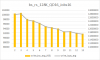Its a bit weird though, while fio clearly shows 10G+ zpool iostat -v is significantly less... I had disabled cache but something is fishy...

Code:
`````` zpool create -f -R /mnt p_sin_str02_v01_o00_cno_sno   gptid/b5c4b9ba-0c2e-11ea-bd58-ac1f6b412042 gptid/b60c9da7-0c2e-11ea-bd58-ac1f6b412042

root@freenas[~]# zfs create p_sin_str02_v01_o00_cno_sno/ds_128k_sync-disabled_compr-off-all && zfs set recordsize=128k sync=disabled compression=off redundant_metadata=all p_sin_str02_v01_o00_cno_sno/ds_128k_sync-disabled_compr-off-all
root@freenas[~]# zfs set primarycache=none p_sin_str02_v01_o00_cno_sno
root@freenas[~]# fio  --direct=1 --refill_buffers --norandommap --randrepeat=0 --group_reporting --ioengine=posixaio --name="p_sin_str02_v01_o00_cno_sno/ds_128k_sync-disabled_compr-off-all"  --runtime=30 --size=100G --time_based  --bs=128k --iodepth=16 --numjobs=16 --rw=write --filename=/mnt/p_sin_str02_v01_o00_cno_sno/ds_128k_sync-disabled_compr-off-all/fio_1.out
p_sin_str02_v01_o00_cno_sno/ds_128k_sync-disabled_compr-off-all: (g=0): rw=write, bs=(R) 128KiB-128KiB, (W) 128KiB-128KiB, (T) 128KiB-128KiB, ioengine=posixaio, iodepth=16
...
fio-3.5
Starting 16 processes
p_sin_str02_v01_o00_cno_sno/ds_128k_sync-disabled_compr-off-all: Laying out IO file (1 file / 102400MiB)
p_sin_str02_v01_o00_cno_sno/ds_128k_sync-disabled_compr-off-all: Laying out IO file (1 file / 102400MiB)
p_sin_str02_v01_o00_cno_sno/ds_128k_sync-disabled_compr-off-all: Laying out IO file (1 file / 102400MiB)
p_sin_str02_v01_o00_cno_sno/ds_128k_sync-disabled_compr-off-all: Laying out IO file (1 file / 102400MiB)
p_sin_str02_v01_o00_cno_sno/ds_128k_sync-disabled_compr-off-all: Laying out IO file (1 file / 102400MiB)
p_sin_str02_v01_o00_cno_sno/ds_128k_sync-disabled_compr-off-all: Laying out IO file (1 file / 102400MiB)
p_sin_str02_v01_o00_cno_sno/ds_128k_sync-disabled_compr-off-all: Laying out IO file (1 file / 102400MiB)
p_sin_str02_v01_o00_cno_sno/ds_128k_sync-disabled_compr-off-all: Laying out IO file (1 file / 102400MiB)
p_sin_str02_v01_o00_cno_sno/ds_128k_sync-disabled_compr-off-all: Laying out IO file (1 file / 102400MiB)
p_sin_str02_v01_o00_cno_sno/ds_128k_sync-disabled_compr-off-all: Laying out IO file (1 file / 102400MiB)
p_sin_str02_v01_o00_cno_sno/ds_128k_sync-disabled_compr-off-all: Laying out IO file (1 file / 102400MiB)
p_sin_str02_v01_o00_cno_sno/ds_128k_sync-disabled_compr-off-all: Laying out IO file (1 file / 102400MiB)
p_sin_str02_v01_o00_cno_sno/ds_128k_sync-disabled_compr-off-all: Laying out IO file (1 file / 102400MiB)
p_sin_str02_v01_o00_cno_sno/ds_128k_sync-disabled_compr-off-all: Laying out IO file (1 file / 102400MiB)
p_sin_str02_v01_o00_cno_sno/ds_128k_sync-disabled_compr-off-all: Laying out IO file (1 file / 102400MiB)
p_sin_str02_v01_o00_cno_sno/ds_128k_sync-disabled_compr-off-all: Laying out IO file (1 file / 102400MiB)
Jobs: 16 (f=16): [W(16)][100.0%][r=0KiB/s,w=15.2GiB/s][r=0,w=125k IOPS][eta 00m:00s]
p_sin_str02_v01_o00_cno_sno/ds_128k_sync-disabled_compr-off-all: (groupid=0, jobs=16): err= 0: pid=36204: Thu Nov 21 18:52:29 2019
write: IOPS=120k, BW=14.7GiB/s (15.7GB/s)(440GiB/30002msec)
slat (nsec): min=759, max=15038k, avg=7886.04, stdev=84017.20
clat (usec): min=17, max=373867, avg=1911.19, stdev=4123.07
lat (usec): min=48, max=373869, avg=1919.08, stdev=4123.37
clat percentiles (usec):
|  1.00th=[   515],  5.00th=[  1074], 10.00th=[  1303], 20.00th=[  1516],
| 30.00th=[  1631], 40.00th=[  1745], 50.00th=[  1827], 60.00th=[  1926],
| 70.00th=[  2024], 80.00th=[  2180], 90.00th=[  2442], 95.00th=[  2704],
| 99.00th=[  3589], 99.50th=[  4113], 99.90th=[  6390], 99.95th=[  7963],
| 99.99th=
bw (  KiB/s): min=401637, max=1077569, per=6.26%, avg=962858.25, stdev=97761.80, samples=958
iops        : min= 3137, max= 8418, avg=7521.90, stdev=763.78, samples=958
lat (usec)   : 20=0.01%, 50=0.02%, 100=0.07%, 250=0.28%, 500=0.58%
lat (usec)   : 750=1.01%, 1000=2.11%
lat (msec)   : 2=63.28%, 4=32.06%, 10=0.54%, 20=0.01%, 50=0.01%
lat (msec)   : 500=0.01%
cpu          : usr=32.12%, sys=7.98%, ctx=1146695, majf=0, minf=32
IO depths    : 1=0.3%, 2=1.4%, 4=5.9%, 8=67.5%, 16=24.8%, 32=0.0%, >=64=0.0%
submit    : 0=0.0%, 4=100.0%, 8=0.0%, 16=0.0%, 32=0.0%, 64=0.0%, >=64=0.0%
complete  : 0=0.0%, 4=93.3%, 8=4.4%, 16=2.3%, 32=0.0%, 64=0.0%, >=64=0.0%
issued rwts: total=0,3604201,0,0 short=0,0,0,0 dropped=0,0,0,0
latency   : target=0, window=0, percentile=100.00%, depth=16

Run status group 0 (all jobs):
WRITE: bw=14.7GiB/s (15.7GB/s), 14.7GiB/s-14.7GiB/s (15.7GB/s-15.7GB/s), io=440GiB (472GB), run=30002-30002msec``````
rerun w longer time
Code:
``````fio  --direct=1 --refill_buffers --norandommap --randrepeat=0 --group_reporting --ioengine=posixaio --name="p_sin_str02_v01_o00_cno_sno/ds_128k_sync-disabled_compr-off-all"  --runtime=60 --size=100G --time_based  --bs=128k --iodepth=16 --numjobs=16 --rw=write --filename=/mnt/p_sin_str02_v01_o00_cno_sno/ds_128k_sync-disabled_compr-off-all/fio_1.out
p_sin_str02_v01_o00_cno_sno/ds_128k_sync-disabled_compr-off-all: (g=0): rw=write, bs=(R) 128KiB-128KiB, (W) 128KiB-128KiB, (T) 128KiB-128KiB, ioengine=posixaio, iodepth=16
...
fio-3.5
Starting 16 processes
p_sin_str02_v01_o00_cno_sno/ds_128k_sync-disabled_compr-off-all: Laying out IO file (1 file / 102400MiB)
p_sin_str02_v01_o00_cno_sno/ds_128k_sync-disabled_compr-off-all: Laying out IO file (1 file / 102400MiB)
p_sin_str02_v01_o00_cno_sno/ds_128k_sync-disabled_compr-off-all: Laying out IO file (1 file / 102400MiB)
p_sin_str02_v01_o00_cno_sno/ds_128k_sync-disabled_compr-off-all: Laying out IO file (1 file / 102400MiB)
p_sin_str02_v01_o00_cno_sno/ds_128k_sync-disabled_compr-off-all: Laying out IO file (1 file / 102400MiB)
p_sin_str02_v01_o00_cno_sno/ds_128k_sync-disabled_compr-off-all: Laying out IO file (1 file / 102400MiB)
p_sin_str02_v01_o00_cno_sno/ds_128k_sync-disabled_compr-off-all: Laying out IO file (1 file / 102400MiB)
p_sin_str02_v01_o00_cno_sno/ds_128k_sync-disabled_compr-off-all: Laying out IO file (1 file / 102400MiB)
p_sin_str02_v01_o00_cno_sno/ds_128k_sync-disabled_compr-off-all: Laying out IO file (1 file / 102400MiB)
p_sin_str02_v01_o00_cno_sno/ds_128k_sync-disabled_compr-off-all: Laying out IO file (1 file / 102400MiB)
p_sin_str02_v01_o00_cno_sno/ds_128k_sync-disabled_compr-off-all: Laying out IO file (1 file / 102400MiB)
p_sin_str02_v01_o00_cno_sno/ds_128k_sync-disabled_compr-off-all: Laying out IO file (1 file / 102400MiB)
p_sin_str02_v01_o00_cno_sno/ds_128k_sync-disabled_compr-off-all: Laying out IO file (1 file / 102400MiB)
p_sin_str02_v01_o00_cno_sno/ds_128k_sync-disabled_compr-off-all: Laying out IO file (1 file / 102400MiB)
p_sin_str02_v01_o00_cno_sno/ds_128k_sync-disabled_compr-off-all: Laying out IO file (1 file / 102400MiB)
p_sin_str02_v01_o00_cno_sno/ds_128k_sync-disabled_compr-off-all: Laying out IO file (1 file / 102400MiB)
Jobs: 16 (f=16): [W(16)][100.0%][r=0KiB/s,w=14.5GiB/s][r=0,w=119k IOPS][eta 00m:00s]
p_sin_str02_v01_o00_cno_sno/ds_128k_sync-disabled_compr-off-all: (groupid=0, jobs=16): err= 0: pid=36369: Thu Nov 21 18:55:46 2019
write: IOPS=114k, BW=13.9GiB/s (14.9GB/s)(834GiB/60002msec)
slat (nsec): min=769, max=21819k, avg=60300.32, stdev=356546.79
clat (usec): min=16, max=24365, avg=1523.24, stdev=1229.34
lat (usec): min=43, max=24441, avg=1583.54, stdev=1268.32
clat percentiles (usec):
|  1.00th=[   72],  5.00th=[  182], 10.00th=[  318], 20.00th=[  594],
| 30.00th=[  865], 40.00th=[ 1156], 50.00th=[ 1434], 60.00th=[ 1663],
| 70.00th=[ 1860], 80.00th=[ 2073], 90.00th=[ 2507], 95.00th=[ 3261],
| 99.00th=[ 6587], 99.50th=[ 8160], 99.90th=, 99.95th=,
| 99.99th=
bw (  KiB/s): min=664064, max=1138865, per=6.26%, avg=912880.15, stdev=87666.37, samples=1920
iops        : min= 5188, max= 8897, avg=7131.48, stdev=684.90, samples=1920
lat (usec)   : 20=0.01%, 50=0.47%, 100=1.44%, 250=5.67%, 500=9.03%
lat (usec)   : 750=9.12%, 1000=9.13%
lat (msec)   : 2=42.09%, 4=19.80%, 10=3.01%, 20=0.23%, 50=0.01%
cpu          : usr=27.02%, sys=34.93%, ctx=5896727, majf=0, minf=32
IO depths    : 1=0.6%, 2=6.2%, 4=15.5%, 8=61.0%, 16=16.6%, 32=0.0%, >=64=0.0%
submit    : 0=0.0%, 4=100.0%, 8=0.0%, 16=0.0%, 32=0.0%, 64=0.0%, >=64=0.0%
complete  : 0=0.0%, 4=93.5%, 8=2.5%, 16=3.9%, 32=0.0%, 64=0.0%, >=64=0.0%
issued rwts: total=0,6832692,0,0 short=0,0,0,0 dropped=0,0,0,0
latency   : target=0, window=0, percentile=100.00%, depth=16

Run status group 0 (all jobs):
WRITE: bw=13.9GiB/s (14.9GB/s), 13.9GiB/s-13.9GiB/s (14.9GB/s-14.9GB/s), io=834GiB (896GB), run=60002-60002msec``````
Code:
``````                                           capacity     operations    bandwidth
--------------------------------------  -----  -----  -----  -----  -----  -----
freenas-boot                            10.4G   139G      0     98      0  1.37M
ada0p2                                10.4G   139G      0     98      0  1.37M
--------------------------------------  -----  -----  -----  -----  -----  -----
p_sin_str02_v01_o00_cno_sno             51.2G  1.40T      0  16.4K      0  2.05G
gptid/b5c4b9ba-0c2e-11ea-bd58-ac1f6b412042  25.6G   714G      0  8.22K      0  1.03G
gptid/b60c9da7-0c2e-11ea-bd58-ac1f6b412042  25.6G   714G      0  8.22K      0  1.03G
--------------------------------------  -----  -----  -----  -----  -----  -----``````

#### gea

##### Well-Known Member
Why have you disabled Arc readcache?
Even on a write test, a lot of metadata must be read what affects even write performance negatively.

#### Rand__

##### Well-Known Member
usually I limit it to 512MB, but I couldn't believe the 14GB/s write speed for a single pair (in a verification run) so I wanted to be sure it wouldnt be caching

Edit: Btw the presentation you referenced sounds quite promising - any time estimate when the goodies will make their way to OmniOS?

#### gea

##### Well-Known Member
You have only disabled readcaching - not writecaching ......

Annual Open-ZFS developper summits show what is in the pipeline with ongoing improvements or new features of Open-ZFS. Some are quite ready when published, others can last.

#### Rand__

##### Well-Known Member
ah that is the result when blindly copying commands instead of checkingThanks,
will check disabling writecache (or set to 512M again to limit impact).
However the other (non verification) results have been run with
vfs.zfs.arc_min: 536870912
vfs.zfs.arc_max: 536870912
vfs.zfs.arc_meta_limit: 536870912

which hopefully is limiting write cache (?)

Edit:
Interestingly 4 nvme drives (2 900p, 2 4800x) do scale better (up to 4 drives I just had at hand) (sync disabled, 128k blocksize/recordsize, stream write, QD16, 16 parallel jobs)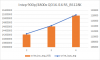Edit2: But not on Qd1 J1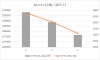Last edited:

#### m4r1k

##### Member
Out of curiosity, have you tried with Solaris? And ZoL?

The reverse scaling might be an Illumos OpenZFS regression. If you have more data, you could actually post on the upstream ZFS community rather than ServeTheHome.

Again, I’m not suggesting you to change your target platform, on contrary, check with different ones may provide additional data to the upstream community that in turns can help them to haunt and fix the issue (especially where DTrace and eBPF are available)

ps: give it a try disabling all the various speculative executions mitigations. At least on Linux, Storage I/O and latency are quite impacted

#### Rand__

##### Well-Known Member
The optane results above were on FreeBSD (FreeNas 11.2U6) so it would be a more general issue than illumos.

Have not tried other platforms but ZoL might be worthwhile, will try to give it a whirl on the weekend.

And yes spectre, meltdown & co caused significant impact to pool performance, but I am not looking to turn the fixes off#### m4r1k

##### Member
The optane results above were on FreeBSD (FreeNas 11.2U6) so it would be a more general issue than illumos.

Have not tried other platforms but ZoL might be worthwhile, will try to give it a whirl on the weekend.

And yes spectre, meltdown & co caused significant impact to pool performance, but I am not looking to turn the fixes offFreeBSD and Illumos share the same ZFS codebase, ZoL has taken a different path. While Solaris is about 10 years of something very different.

All my suggestions is to help with the RCA, if you identify a working system upstream can target it to fix their. Then up to you what to test

#### Rand__

##### Well-Known Member
All my suggestions is to help with the RCA, if you identify a working system upstream can target it to fix their. Then up to you what to test
Appreciate itBuilt the latest ZoL from github on an Centos 7.7 box.

2 disk stripe optane pool, 16/16/128 (sync disabled) (no provisions to turn of cache and the box has plenty of ram)

Run status group 0 (all jobs):
WRITE: bw=22.8GiB/s (24.5GB/s), 22.8GiB/s-22.8GiB/s (24.5GB/s-24.5GB/s), io=684GiB (734GB), run=30002-30002msec

Same with QD1/J1
Run status group 0 (all jobs):
WRITE: bw=2049MiB/s (2149MB/s), 2049MiB/s-2049MiB/s (2149MB/s-2149MB/s), io=60.0GiB (64.5GB), run=30001-30001msec

With sync=always
16/16
Run status group 0 (all jobs):
WRITE: bw=2720MiB/s (2852MB/s), 2720MiB/s-2720MiB/s (2852MB/s-2852MB/s), io=79.7GiB (85.6GB), run=30012-30012msec

Same with QD1/J1
Run status group 0 (all jobs):
WRITE: bw=553MiB/s (580MB/s), 553MiB/s-553MiB/s (580MB/s-580MB/s), io=16.2GiB (17.4GB), run=30001-30001msec

Same with stripe of 4

p_sin_str02_v01_o00_cno_sno ONLINE 0 0 0
nvme0n1 ONLINE 0 0 0
nvme3n1 ONLINE 0 0 0
nvme1n1 ONLINE 0 0 0
nvme2n1 ONLINE 0 0 0

sync disabled
16/16
Run status group 0 (all jobs):
WRITE: bw=17.7GiB/s (18.0GB/s), 17.7GiB/s-17.7GiB/s (18.0GB/s-18.0GB/s), io=531GiB (570GB), run=30002-30002msec

Same with QD1/J1
Run status group 0 (all jobs):
WRITE: bw=2057MiB/s (2157MB/s), 2057MiB/s-2057MiB/s (2157MB/s-2157MB/s), io=60.3GiB (64.7GB), run=30001-30001msec

Single Device
16/16
Run status group 0 (all jobs):
WRITE: bw=20.6GiB/s (22.1GB/s), 20.6GiB/s-20.6GiB/s (22.1GB/s-22.1GB/s), io=617GiB (662GB), run=30003-30003msec

1/1
Run status group 0 (all jobs):
WRITE: bw=1474MiB/s (1546MB/s), 1474MiB/s-1474MiB/s (1546MB/s-1546MB/s), io=43.2GiB (46.4GB), run=30001-30001msec

1/1 sync always
Run status group 0 (all jobs):
WRITE: bw=570MiB/s (598MB/s), 570MiB/s-570MiB/s (598MB/s-598MB/s), io=16.7GiB (17.9GB), run=30001-30001msec

5 min runtime just to be sure that its not only cache speed
1/1, sync
Run status group 0 (all jobs):
WRITE: bw=585MiB/s (613MB/s), 585MiB/s-585MiB/s (613MB/s-613MB/s), io=171GiB (184GB), run=300001-300001msec

16/16, sync
Run status group 0 (all jobs):
WRITE: bw=1511MiB/s (1584MB/s), 1511MiB/s-1511MiB/s (1584MB/s-1584MB/s), io=443GiB (475GB), run=300020-300020msec

So where does that leave us?
Single device performance (at qd1/j1) is at expected level. Second device adds 30% on top and anything else is basically wasted at this time.

So strategy for me has to be 'Get a pair (or 4 for mirror pairs) of the fastest/biggest drives I can get/afford' and call it a day...

edit - just for good measure
1/1 on single device with sync always and pmem slog
Run status group 0 (all jobs):
WRITE: bw=947MiB/s (993MB/s), 947MiB/s-947MiB/s (993MB/s-993MB/s), io=27.7GiB (29.8GB), run=30001-30001msec

does not scale with more devices (as without slog)

16/16
un status group 0 (all jobs):
WRITE: bw=2499MiB/s (2621MB/s), 2499MiB/s-2499MiB/s (2621MB/s-2621MB/s), io=73.2GiB (78.6GB), run=30007-30007msec

does scale some with 4 devices

Run status group 0 (all jobs):
WRITE: bw=3848MiB/s (4035MB/s), 3848MiB/s-3848MiB/s (4035MB/s-4035MB/s), io=113GiB (121GB), run=30008-30008msec

Last edited: